New Students Offer - Use Code HELLO

# Syllogism Rules with Examples PDF

Syllogism is a very important topic for exams. It is also referred as 'Logic'. Here given tips and tricks to solve these questions easily.### Proposition

Proposition is also referred as ‘Logic’. It is a sentence, that asserts that either a part of or the whole of, one set of objects- the set identified by the subjects term in the sentence expressing that sentence either is included in or is excluded from, another set- the set identified by the predicate term in that sentence.### Parts of Proposition

It consists four parts.
1.      Quantifier- In quantifier, the words ‘all’, ‘no’ and ‘some’ are used as they express quantity. ‘All’ and ‘no’ are universal quantifiers because they refer to every object in a certain set and quantifier ‘some’ is a particular quantifier because it refers to atleast one existing one existing object in a certain set.

2.      Subject- It is the word about which something is said.

3.      Predicate- It is the part of proposition which denotes which is affirmed or denied about the subject.

4.      Copula- It is the part of proposition which denotes the relation between the subject and predicate.

Example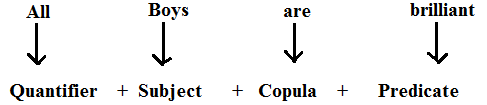Hence, the standard form of proposition is
Quantifier + Subject + Copula + Predicate

#### Four-fold Classification of Categorical Proposition

On the basis of quality of proposition we can classify them in four categories. To draw valid inferences, it is necessary to have a clear understanding of the A, E, I, O relationship as given in the table.
 Symbol Proposition Quantity Quality AEIO All A are BNo A is BSome A are BSome A are not B UniversalUniversalParticularParticular AffirmativeNegativeAffirmativeNegative

### Rules for Deriving the Conclusions from Two Given Premises

1.      Universal Affirmative or A-type Proposition
Take an example: All goas are dogs.
This is A-type proposition. We can see it by graphical representation of the above Proposition we observe that goats are distributed in dogs. Hence, we can conclude that in A-type proposition only subject is distributed.2.      Universal Negative or E-type Proposition
Take an example: No girl is boy.
In this type of proposition, both subject and predicate are denial of each other. This can also be seen in the diagram representing boy and girl. They have nothing in common. Hence, both subject and predicate are distributed.3.      Particular Affirmative or I-type Proposition
Take an example: Some mobiles are telephones.
In this type of proposition, subject and predicate have something in common. This implies that in I-type neither subject nor predicate is distributed. We can see it graphically as given in figure.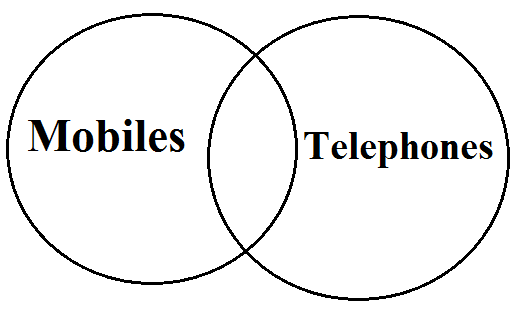4.      Particular Negative or O-Type Proposition
Take an example: Some boys are not students.
In O-type propositions, some of the category represented by boys subjects, which means that a section of boys is denied with the entire category of students. It is, therefore, deduced that in O-type proposition only predicate is distributed. On account of different logical approach required to be applied drawing each type of inference, a clear understanding of this difference becomes more important.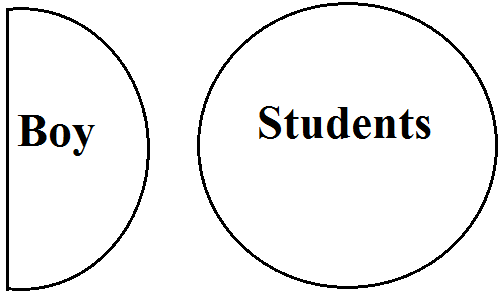### Rules for Mediate Inference

First introduced by Aristotle, a syllogism is a deductive argument in which conclusion has to be drawn from two propositions referred to as premises.
Now consider as example
Statements
Vinay is a boy.
All boys are honest.
Conclusion I. Vinay is honest.
First two sentences and are called propositions and the sentence I is called conclusion. This conclusion is drawn from above given two propositions.

Type of Questions Asked in the Examination There are mainly two types of questions which have been asked in various Bank PO examinations.
1.      When Premises are in specified Form
Here, premise is in specified form. Here, mainly two propositions are given. Propositions may be particular to universal; universal to particular; particular to particular; universal to universal.
2.      When premises are in Jumbled/Mixed Form
Here, at least, three or more than three proposition are given. Here, pair of two propositions out of them follow as same as in specified form.

## Type 1. Specified Form Problems

### Case 1. The conclusion does not contain the middle term.

#### Example 1.

Statements All men are girls.
Some girls are students.
Conclusions I. All girls are men.
II. Some girls are not students.
Solution. Since both the Conclusion I and II contain the middle term ‘girls’ so neither of them can follow.

Venn Diagram Representation All possible  cases can be drawn by using venn diagram.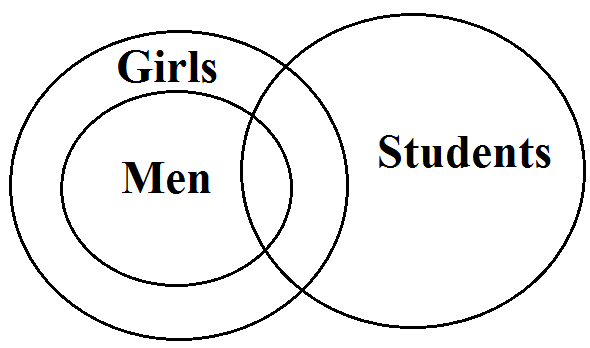(a)(b)

By using both representation (a) and (b), it is clear all girls cannot be men as well as (a) shows some girls are students, here no man is included but at the same time (b) shows some girls are students, here some men are also students as all men are girls. Hence, we cannot deduce Conclusion II. So, neither of them can follow.

### Case 2. No term can be distributed in the conclusion unless it is distributed in the premises.

#### Example 2.

Statements Some boys are students.
All students are teenagers.
Conclusions I. All teenagers are students
II. Some boys are teenagers.
Solution. First statement is an I-type proposition which distributes neither the subject nor the predicate. Second Statement II is an A-type proposition which distributes the subject ‘students’. Conclusion I is an A-type propositions which distributes the subject ‘teenager’ only.
Since, the term teenagers is distributed in Conclusion I without being distributed in the premises. So, Conclusion I cannot follows. In second conclusion, where it is asked that some boys are teenagers. But from first statement, it is clear some students are not boys. These students may not be teenagers.
Venn Diagram Representation All possible cases be drawn as follows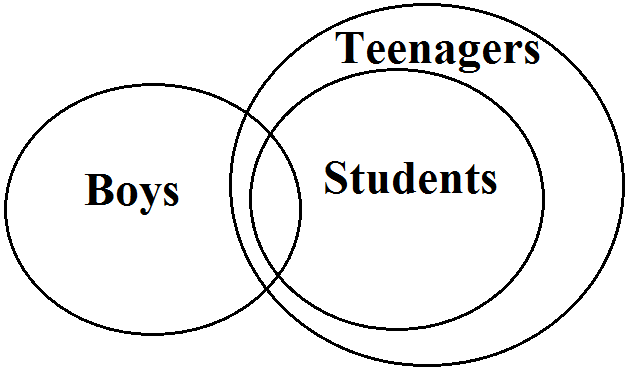We have given all students are teenagers, so its reverse cannot be possible. Hence, conclusion I is false. As we are also given some boys are students and all students are teenagers. So, some boys which are students must be teenagers. Hence, Conclusion II follows.

### Case 3. If one premises is particular, conclusion is particular.

#### Example 3.

Statements Some boys are thieves.
All thieves are dacoits.
Conclusions I. Some boys are dacoits.
II. All dacoits are boys.
Solution. Since one premise is particular, the conclusion must be particular,so Conclusion II cannot follows.
Venn Diagram Representation All possible cases can be drawn as followsHere, conclusion I follows but the Conclusion II cannot follows.

### Case 4. If the middle term is distributed twice, the conclusion cannot be universal.

#### Example 4.

Statements All Lotus are flowers.
No Lily is a Lotus.
Conclusions I. No Lily is flowers.
II. Some Lilies are flowers.
Solution. Here, the first premise is an A-type proposition and so, the middle term ‘Lotus’ forming the predicate is distributed. Since, the middle term is distributed twice, so the conclusion cannot be universal.
Venn Diagram Representation All possible cases can be drawn as follows.(a)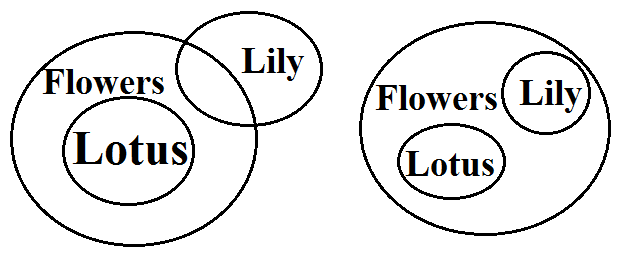(b)                      (c)

It is clear from the given venn diagrams, either Conclusion I or II must be followed.

### Case 5. If both the premises are affirmative, the Conclusion must be followed.

#### Example 5.

Statements All garden are schools.
All schools are colleges.
Conclusions I. All gardens are colleges.
II. Some gardens are not colleges.
Solution. Since, both the premises are affirmative, the conclusion must be affirmative, the conclusion II cannot follows.
Venn Diagram Representation All possible cases can be drawn as follows.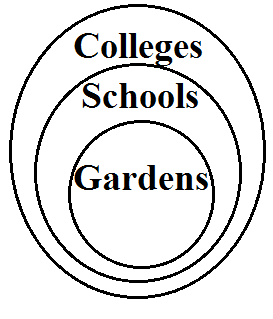Now taking Conclusion I, it is clear all gardens are also colleges. But taking Conclusion II, we cannot derive second conclusion is true. Hence, only first conclusion must be true.

### Case 6. No conclusion follows.

There are three types of such cases.
a)     If both the premises are particular.

#### Example 6.

Statements Some cups are spoons.
Some spoons are saucers.
Solution. Since, both the premises are particular, so no definite conclusion follows.
Venn Diagram Representation(a)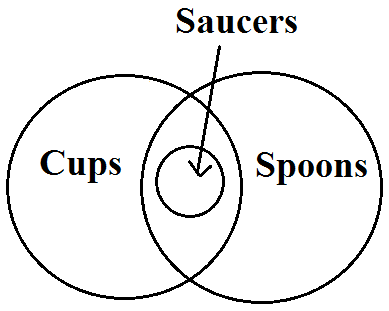(b)

It is clear from both given venn diagrams that no conclusion is followed.

b)     It both the premises are negative.

#### Example 7.

Statements No flower is mango.
No mango is cherry.
Conclusions I. No flower is cherry.
II. Some cherries are mangoes.
Solution. Since, both the premises are negative, hence neither conclusion follows.
Venn Diagram Representation(a)(b)

It is clear from both venn diagrams that neither conclusion follows.

c)     If the major premise is particular and the minor premise is negative. Or Major premise is the predicate of the conclusion and minor premise is the subject of the conclusion.

#### Example 8.

Statements Some pups are cows.
No kittens are pups.
Conclusions I. No pups are kitten.
II. Some Cows are kitten.
Solution. Here, the first premise containing the middle term ‘kitten’ as the subject is the major premise and the second premise containing the middle terms ‘kitten’ as the predicate is the minor premise. Since, the major promise is particular and the minor premise is negative. So, no conclusion follows.

Venn Diagram Representation All possible cases are given.(a)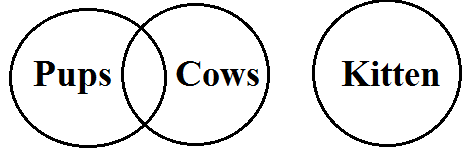(b)
It is clear from the venn diagram representation, none conclusion follows.

### Complementary Pair of Conclusions

In drawing mediate inferences from given statements, students are required to be more attentive to select complementary pair of conclusion where neither of the conclusions is definitely true but a combination of both makes a complementary pair. As we have already discussed in Case 1 that in the statements where middle term is not distributed, no valid mediate inference can be drawn but there still exists a possibility that a complimentary pair of conclusions follows from the statements.

#### Example 9.

Some statues are cameras.
Conclusions I. Some radios are statues.
Solution. Either “some radios are statues” or “no radio is statue”, as I and E-type propositions form a complementary pair.
Venn Diagram Representation We can draw all possible cases as given below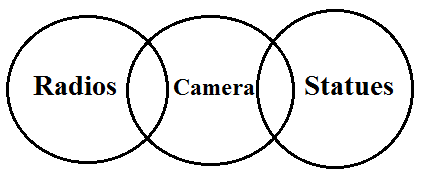(a)(b)
Hence using both diagrammatically representation, we can conclude either ‘some radios are statues’ or ‘no radio is statue.’ Hence, atleast, one of the conclusions must be true.

### Special Cases

Facts

 Combination (Conclusion) Conclusion A + OE + II + O Either I or II followsEither I or II followsEither I or II follows

Example 10. Statements All vegetables are green.
Some greens are fruits.
Conclusions  I. Some fruits are vegetables.
II. No fruit is vegetable.
Solution. Here, Conclusion I is particular affirmative and Conclusion II is universal negative proposition. Hence, either Conclusion I or Conclusion II follows.(a)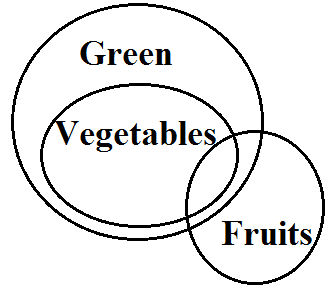(b)

Conclusion If we follow venn diagram (a), then we can say no fruit  is vegetable Conclusion II but if we follow venn diagram (b), then we can say some fruits are vegetables. (Conclusion I)
Here either venn diagram (a) or venn diagram (b) is possible. Hence, Conclusion I or Conclusion II must be followed.

Minimal Possibilities We can represent statements by keeping in mind one conclusions. If we follow that our two conclusions belong to special case, then either one of them is true.

#### Example 11.

Statements Some fruits are vegetables.
Some vegetables are junk food.
Conclusions I. Some junk foods are vegetables.
II. Some junk food are fruits.
Solution.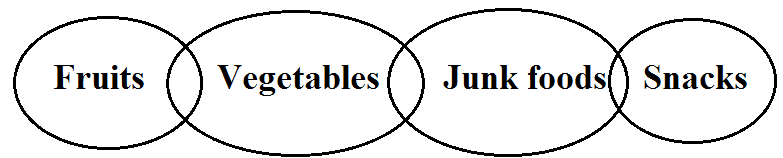It is clear the above diagram that only Conclusion I follows.

Possibility.
Possibility is a concept of inconsistency for an event which is not yet verified but if true would explain certain facts or phenomena. In other words capability of existing or happening or being true known as possibility
 Condition Possibility Given factsImaginary facts Cannot be determinedCan be determined
It is so simple to understand through the above table that possibility exists where no definite relation occurs between the objects and definite relation between the objects eliminate existence of any possibility. In simple way given with an example which will also clear the term possibility.

#### Example 12.

Statements Some birds are trees.
Some trees are hens.
Conclusions I. Some birds being hens is a possibility.
II. All trees being hens is a possibility.
Solution. In Conclusion I, before deciding the possibility between birds and hens, we must notice the relation between both, we find that there is no relation between birds and hens, so possibility favours the condition and the Conclusion I is true for possibility and in Conclusion II we must notice the relation between trees and hens. We find that both have some type of relation between them so the possibility of ‘All’ between trees and hens is true. Hence, both the Conclusions I and II follows.
 Given Exclusive Proportion Desired Proportions Possibility AllSomeNoNoSomeNo proper relations AllSomeNoSome notAllSome All NoNoNoNoYesYes

Note Improper relation between two objects favours the possibility (In above example Conclusion I)

Special Cases of Exclusive Proposition
 If the statement is of Conversion Illustration Meaningful Conversion Much, more, many, very, a few, most, almostAtleastDefinitely Only1% to 99% Some SomeNo use Some Most A are B.A few X are Y.Atleast some A are B.Some A are definitely B.Some X are definitely not Y.Only A are B.38% A are B.98% X are Y. Some A are B.Some X or Y.Some A are B.Some A are B.Some X are not Y.All B are A.Some A are B.Some X are Y.

### Memorable Points

1. Always positive conclusion comes from positive statements.
2. Always negative conclusion comes from negative statements.
3. Some type conclusion will always be formed form all type statement.
4. If two statements are joined together, ‘some’ type conclusion will definitely come out. Either the relation between statement is ‘all’ type or ‘some’ type.
5. Generally a negative conclusion does not come out from a positive statement.
6. Generally a positive conclusion does not come out from a negative statement.
For any query, Kindly Comment.